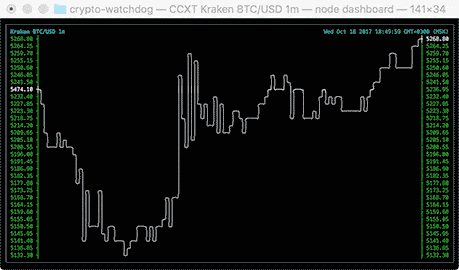# kroitor/asciichart

Nice-looking lightweight console ASCII line charts ╭┈╯ for NodeJS, browsers and terminal, no dependencies
Python JavaScript HTML
Latest commit cf6bdb8 Nov 11, 2019
Type Name Latest commit message Commit time
Failed to load latest commit information.asciichartpy Jun 24, 2019.eslintrc Dec 10, 2017.gitattributes Jul 21, 2017.gitignore Jun 17, 2019.npmignore Jun 24, 2019.travis.yml Jul 21, 2018CHANGELOG.md Jul 21, 2017LICENSE.txt Sep 20, 2017MANIFEST.in Sep 2, 2017README.md Nov 11, 2019README.rst Sep 2, 2017asciichart.js Jun 17, 2019asciichart.sublime-project Sep 1, 2017index.html Feb 10, 2017package.json Jun 17, 2019pplot Jun 24, 2019setup.cfg Sep 2, 2017setup.py Jun 24, 2019test.js Jun 17, 2019test.py Sep 2, 2017tox.ini Sep 2, 2017

# asciichart

Console ASCII line charts in pure Javascript (for NodeJS and browsers) with no dependencies. This code is absolutely free for any usage, you just do whatever the fuck you want.## Usage

### NodeJS

`npm install asciichart`
```var asciichart = require ('asciichart')
var s0 = new Array (120)
for (var i = 0; i < s0.length; i++)
s0[i] = 15 * Math.sin (i * ((Math.PI * 4) / s0.length))
console.log (asciichart.plot (s0))```

### Browsers

```<!DOCTYPE html>
<html>
<meta http-equiv="Content-Type" content="text/html; charset=utf-8">
<meta charset="UTF-8">
<title>asciichart</title>
<script src="asciichart.js"></script>
<script type="text/javascript">
var s0 = new Array (120)
for (var i = 0; i < s0.length; i++)
s0[i] = 15 * Math.sin (i * ((Math.PI * 4) / s0.length))
console.log (asciichart.plot (s0))
</script>
<body>
</body>
</html>```

### Options

The width of the chart will always equal the length of data series. The height and range are determined automatically.

```var s0 = new Array (120)
for (var i = 0; i < s0.length; i++)
s0[i] = 15 * Math.sin (i * ((Math.PI * 4) / s0.length))
console.log (asciichart.plot (s0))```The output can be configured by passing a second parameter to the `plot (series, config)` function. The following options are supported:

```var config = {

offset:  3,          // axis offset from the left (min 2)
padding: '       ',  // padding string for label formatting (can be overrided)
height:  10,         // any height you want

// the label format function applies default padding
format:  function (x, i) { return (padding + x.toFixed (2)).slice (-padding.length) }
}```

### Scale To Desired Height```var s = []
for (var i = 0; i < 120; i++)
s[i] = 15 * Math.cos (i * ((Math.PI * 8) / 120)) // values range from -15 to +15
console.log (asciichart.plot (s, { height: 6 }))     // this rescales the graph to ±3 lines```### Auto-range

```var s2 = new Array (120)
s2 = Math.round (Math.random () * 15)
for (i = 1; i < s2.length; i++)
s2[i] = s2[i - 1] + Math.round (Math.random () * (Math.random () > 0.5 ? 2 : -2))
console.log (asciichart.plot (s2))```A util by madnight for drawing Bitcoin/Ether/altcoin charts in command-line console: bitcoin-chart-cli.### Ports

Special thx to all who helped port it to other languages, great stuff!

### Future work (coming soon, hopefully)

• multi-line colored charts in console!
• levels and points on the graph!
• even better value formatting and auto-scaling!You can’t perform that action at this time.